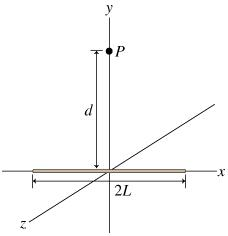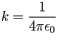# Problem: A charged wire of negligible thickness has length 2L units and has a linear charge density lambda. Consider the electric field E-vector at the point P, a distance d above the midpoint of the wire. The field E-vector points along one of the primary axes, yWhat is the magnitude E of the electric field at point P? Throughout this part, express your answers in terms of the constant k, defined by

###### FREE Expert Solution
95% (344 ratings)View Complete Written Solution
###### Problem DetailsA charged wire of negligible thickness has length 2L units and has a linear charge density lambda. Consider the electric field E-vector at the point P, a distance d above the midpoint of the wire. The field E-vector points along one of the primary axes, y

What is the magnitude E of the electric field at point P? Throughout this part, express your answers in terms of the constant k, defined by